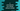# C program to concatenate two strings without using strcat()### C program to concatenate two strings without using strcat() :

In this tutorial, we will learn how to concatenate two strings without using strcat() function in C programming language. We will take the input from the user. User will enter both strings, our program will concatenate the strings and print out the result. To store the strings, we will use two arrays. To read the strings, we will use gets() function. Let’s take a look into the program first :

### C program :

``````#include <stdio.h>
#include <stdlib.h>

int main()
{
//1
char firstString, secondString;

//2
int i, j;

//3
printf("Enter your first string : ");

//4
gets(firstString);

//5
printf("Enter your second string : ");

//6
gets(secondString);

//7
i = 0;

//8
while (firstString[i] != '\0')
{
i++;
}

//9
j = 0;

//10
while (secondString[j] != '\0')
{
firstString[i] = secondString[j];
i++;
j++;
}

//11
firstString[i] = '\0';

//12
printf("Final String : %s\n", firstString);

return 0;
}
``````

### Explanation :

The commented numbers in the above program denotes the step number below :

1. Create two char array variables to store the strings. These variables are firstString and secondString. The size of these arrays are 100.
2. Create two integer variables i and j to store the current position of index for firstString and secondString.
3. Ask the user to enter the first string.
4. Store it on variable firstString. We are reading the string using gets() .
5. Ask the user to enter the second string.
6. Store the string in secondString variable.
7. Initialize the variable i as 0. This variable will indicate the current index for the firstString.
8. Using one while loop, move the value of i to the last position of the string. e.g. if the string is hello, the i value after while loop will be 5.
9. Initialize j as 0. This variable will indicate the current index for secondString.
10. Using a while loop, read the secondString character by character and append each character to firstString. Since, i is pointing to the end of initial firstString, all the values of secondString will be append to the end of firstString.
11. After the while loop is completed, add one end of string ‘\0’ character to the end of firstString.
12. Print the final String. Final string is saved on firstString variable.

### Example Output :

``````Enter your first string : This is
Enter your second string : a book
Final String : This isa book

Enter your first string : This is
Enter your second string :  a cat
Final String : This is a cat

Enter your first string : 1,2,3,4,5,
Enter your second string : 6,7,8,9,10
Final String : 1,2,3,4,5,6,7,8,9,10
``````